# Introduction

The early stages of vision processing identify features in images that are relevant to estimating the structure and properties of objects in a scene. An edge in an image is a significant local change in the image intensity, usually associated with a discontinuity in either the image intensity or the first derivative of the image intensity. They are important features for analyzing images. They occur at the boundary between two different regions in an image. It is the first step in recovering information from images.

Discontinuities in the image intensity can be either (1) step discontinuities, where the image intensity abruptly changes from one value on one side of the discontinuity to a different value on the opposite side, or (2) line discontinuities, where the image intensity abruptly changes value but then returns to the starting value within some short distance. However, step and line edges are rare in real images. Because of low-frequency components or the smoothing introduced by most sensing devices, sharp discontinuities rarely exist in real signals. Step edges become ramp edges and line edges become roof edges, where intensity changes are not instantaneous but occur over a finite distance.

Reference: MachineVision_Chapter5.pdf (usf.edu)

General Steps In Edge Detection:

Filter: We may encounter noise while computing gradients that needs removal for accurate edge detection. However, there is a tradeoff between edge strength and noise reduction.

Detection: We only want points with strong edge content. However, many points in an image have a nonzero value for the gradient, and not all of these points are edges for a particular application. Thresholding provides the criterion used for detection.

Enhancement: This step involves emphasizing pixels where significant change in local neighbourhood, involves gradient and magnitude calculation.

Common Methodologies: Canny edge detector, Sobel edge detector, Prewitt edge detector, Laplacian edge detector

Assumption: Convert to grayscale and normalize pixel intensity between 0 to 255.

# Canny edge detector: Theory

Canny operator is a multi-stage algorithm that detects wide range of edges.

The Canny edge detection algorithm is composed of 5 steps:

1. Noise reduction: One way to get rid of the noise on the image, is by applying Gaussian blur to smooth it. To do so, image convolution technique is applied with a Gaussian Kernel (3x3, 5x5, 7x7 are common). The kernel size depends on the expected blurring effect. Basically, the smallest the kernel, the less visible is the blur. In our example, we will use a 5 by 5 Gaussian kernel. The equation for a Gaussian filter kernel of size (2k+1)×(2k+1) is given by:

2. Gradient calculation: The Gradient calculation step detects the edge intensity and direction by calculating the gradient of the image using edge detection operators. Edges correspond to a change of pixels’ intensity. To detect it, the easiest way is to apply filters that highlight this intensity change in both directions: horizontal (x) and vertical (y). When the image is smoothed, the derivatives in both directions calculated by convolving with Sobel kernels.

3. Non-maximum suppression: Ideally, the final image should have thin edges. Thus, we must perform non-maximum suppression to thin out the edges. The algorithm goes through all the points on the gradient intensity matrix and finds the pixels with the maximum value in the edge directions.

4. Double threshold: Two thresholds for pixel intensities.

• High threshold: Pixel intensities beyond this threshold are edges
• Low threshold: Pixel intensities below this threshold are not edges
• All pixels having intensity between both thresholds are flagged as weak and the Hysteresis mechanism (next step) will help us identify the ones that could be considered as strong and the ones that are considered as non-relevant.

5. Hysteresis: This step transforms weak pixels between both thresholds into strong ones if and only if at least one of the pixels around the weak pixel being processed is a strong one using connectivity analysis as shown below.

Pros: Immune to noisy environment due to strong smoothing effect, enhances signal to noise ratio, good localisation and response.

Cons: Computationally expensive such that real time implementation is difficult.

Additional Points: An adaptive edge-detection algorithm is necessary to provide a robust solution that is adaptable to the varying noise levels of these images to help distinguish valid image contents from visual artifacts introduced by noise. The performance of the Canny algorithm depends heavily on common adjustable parameters like standard deviation σ for the Gaussian filter and the threshold values as well. The bigger the value for σ, the larger the size of the Gaussian filter becomes. This implies more blurring, which is necessary for noisy images, as well as detecting larger edges. However, localization of the edge becomes less. Smaller values of σ limits blurring but maintain finer edges in the image. The user can tailor the algorithm by adjusting these parameters to adapt to different environments.

# Sobel Edge Detector: Theory

1. Firstly, convert the image from an RGB scale to a Grayscale image. Then from there, we will use what is called kernel convolution. There are two kernels used for convolution with original: one in horizontal x direction and other in vertical y direction.
2. When we want to scan across the X direction of an image for example, we will want to use the following X Direction Kernel to scan for large changes in the gradient. Similarly, when we want to scan across the Y direction of an image, we could also use the following Y Direction Kernel to scan for large gradients as well.

It is based on the fact that in the edge area, the pixel intensity shows a “jump” or a high variation of intensity.

Pros: Simple and easy to implement.

Cons: There tends to be a lot of noise in the final processed image. As you can see in the image above. Use an averaging or median filter to smoothen the image, and then apply the Sobel Edge Detection Algorithm again and compare the differences. It is not as powerful as Canny Edge detector.

# Prewitt edge detector: Theory

Resource: Prewitt Operator — Tutorialspoint

This operator does not place any emphasis on the pixels that are closer to the center of the mask.

Prewitt detects two types of edges

• Horizontal edges
• Vertical Edges

Edges are calculated by using difference between corresponding pixel intensities of an image.

All the derivative masks should have the following properties:

• Opposite sign should be present in the mask.
• Sum of mask should be equal to zero.
• More weight means more edge detection.

# Vertical direction

The zeros column in the vertical direction. When you will convolve this mask on an image, it will give you the vertical edges in an image. It calculates the difference of pixel intensities in a edge region.

Center column is zero and not included. Only the difference between right and left pixel values around that edge are computed. This increase the edge intensity and it become enhanced comparatively to the original image.

# Horizontal Direction

Zeros column is in horizontal direction. When you will convolve this mask onto an image it would horizontal edges in the image.

It also calculates difference among the pixel intensities of a particular edge. As the center row of mask is consist of zeros so it does not include the original values of edge in the image but rather it calculate the difference of above and below pixel intensities of the particular edge. Thus increasing the sudden change of intensities and making the edge more visible.

# Sample Image

Following is a sample picture on which we will apply above two masks one at time.

Resource: Prewitt Operator — Tutorialspoint

Pros: Simple ability to detect edge and orientation.

Cons: Inaccurate and highly sensitive to noise

# Second Order Methods

All of the previous edge detectors have approximated the first order derivatives of pixel values in an image. It is also possible to use second order derivatives to detect edges. A very popular second order operator is the Laplacian operator.

The Laplacian of a function f(x,y), denoted by

It is defined by:

Once more we can use discrete difference approximations to estimate the derivatives and represent the Laplacian operator with the

Disadvantages to the use of second order derivatives:

• Second derivatives will exaggerated noise twice as much as first order and gradient based ones
• No directional information about the edge is given.

# Laplacian Edge Detector: Theory

Laplacian Operator is also a derivative operator which is used to find edges in an image. The major difference between Laplacian and other operators like Prewitt, Sobel, Robinson and Kirsch is that these all are first order derivative masks but Laplacian is a second order derivative mask. In this mask we have two further classifications one is Positive Laplacian Operator and other is Negative Laplacian Operator.

Laplacian didn’t take out edges in any particular direction but it take out edges in following classification.

• Inward Edges
• Outward Edges

Let’s see that how Laplacian operator works.

# Positive Laplacian Operator

In Positive Laplacian we have standard mask in which center element of the mask should be negative and corner elements of mask should be zero.

Positive Laplacian Operator is use to take out outward edges in an image.

# Negative Laplacian Operator

In negative Laplacian operator we also have a standard mask, in which center element should be positive. All the elements in the corner should be zero and rest of all the elements in the mask should be -1.

Negative Laplacian operator is use to take out inward edges in an image.

# Steps:

Laplacian is a derivative operator; its uses t gray level discontinuities in an image and try to deemphasize regions with slowly varying gray levels. This operation in result produces such images which have grayish edge lines and other discontinuities on a dark background. This produces inward and outward edges in an image

# After applying Positive Laplacian Operator

If we apply positive Laplacian operator on the image then we subtract the resultant image from the original image to get the sharpened image.

# After applying Negative Laplacian Operator

Similarly if we apply negative Laplacian operator then we have to add the resultant image onto original image to get the sharpened image.

# Takeaway:

Canny Edge Detection algorithm is computationally more expensive compared to Sobel, Prewitt and Laplacian operator. However, the Canny’s edge detection algorithm performs better than all these operators under almost all scenarios, even under noisy conditions.

--

--

--

Passionate about data science and engineering, python programming and database management

Love podcasts or audiobooks? Learn on the go with our new app.

## TOD-BERT: Pre-training Transformer for Task-Oriented Dialogue Systems## What’s so hard about ordering blueberries?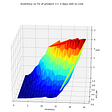## How to Train Yolo v3 to Detect Custom Objects with Free GPU## ML Classifier Performance Comparison for Spam Emails Detection (Part 2)## ML Classification Metrics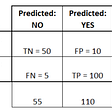## pytorch-widedeep: deep learning for tabular data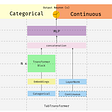## Image to image translation using BicycleGAN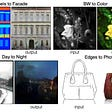## Anirban Kar Chaudhuri

Passionate about data science and engineering, python programming and database management

## Finding the Duplicate Number using Floyd’s Tortoise and Hare Algorithm## Machine Learning Based Thread Pool Tuning via Program Analysis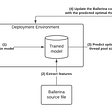## Role of mathematics in image processing